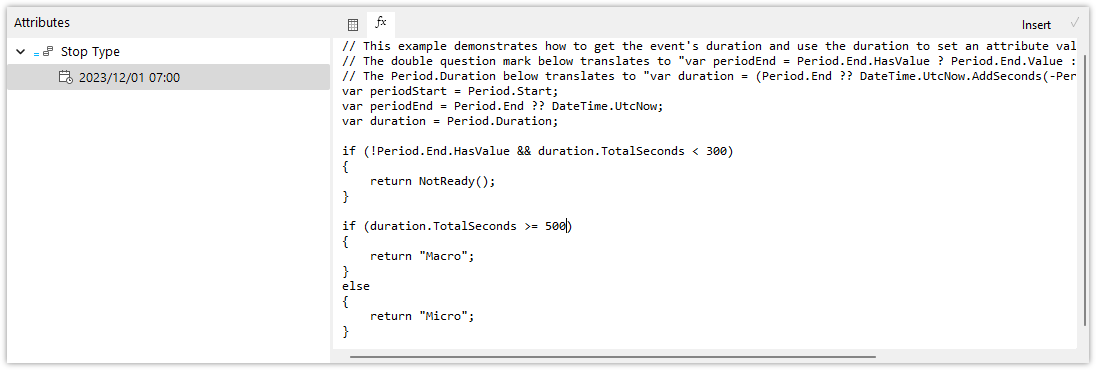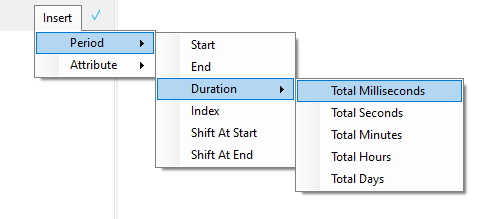Follow

Using Attributes - Calculated Attributes

This article will be updated in the near future! In the meanwhile, if the following information is insufficient, contact support@flow-software.com.

It is possible to add one or more Calculated Attributes to your Event.Similar to Calculated Measures, a calculated attribute allows you to configure multiple calculations, which are identified by a date & time.  This is the time from which the calculation is valid.

There are a few built-in properties that you can use in your calculation.  These maybe inserted by selecting the Insert menu on the top-right of the calculation editor.Period > Start Returns the UTC timestamp when the event period began (when the start trigger occurred), represented as Period.Start in the calculation. Period > End Returns the UTC timestamp when the event period ended (when the end trigger occurred), represented as Period.End in the calculation Duration > Total Milliseconds Returns the duration of the event in milliseconds (as a floating point number). This can only be evaluated when the event has ended. This is represented as (Period.End-Period.Start).TotalMilliseconds in the calculation Duration > Total Seconds Returns the duration of the event in seconds (as a floating point number). This can only be evaluated when the event has ended. This is represented as (Period.End-Period.Start).TotalSeconds in the calculation Duration > Total Minutes Returns the duration of the event in minutes (as a floating point number). This can only be evaluated when the event has ended. This is represented as (Period.End-Period.Start).TotalMinutes in the calculation Duration > Total Hours Returns the duration of the event in hours (as a floating point number). This can only be evaluated when the event has ended. This is represented as (Period.End - Period.Start).TotalHours in the calculation Duration > Total Days Returns the duration of the event in days (as a floating point number). This can only be evaluated when the event has ended. This is represented as (Period.End-Period.Start).TotalDays in the calculation

Calculations must be validated before they can be used.  Validation involves Flow checking that the calculation you've written is valid C# code and can be processed by the Flow Engine.  To validate your calculation, select the green "Check" button in the top right of the calculation editor.